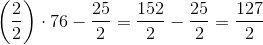# PSAT Math : How to subtract complex fractions

## Example Questions

### Example Question #2 : Complex Fractions

Solve: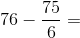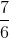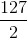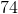Explanation:

First reduce the fraction. We can divide both the numerator and the denominator by 3.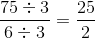Now our expression looks like this: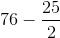When you add or subtract fractions, you need to have the same denominator. The lowest common deonminator here is 2. So we need to multiply and solve: